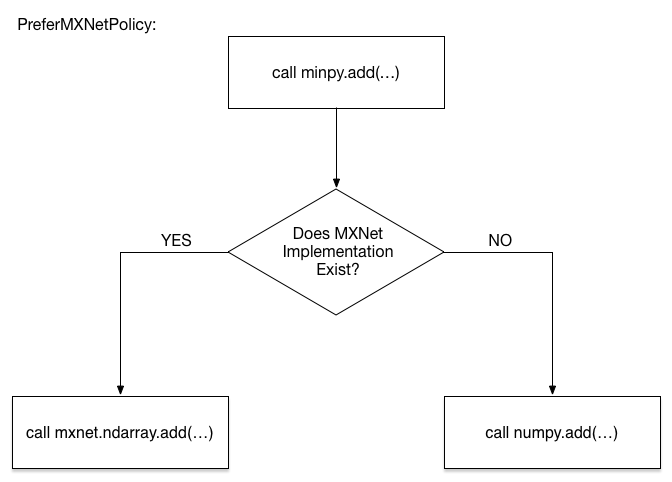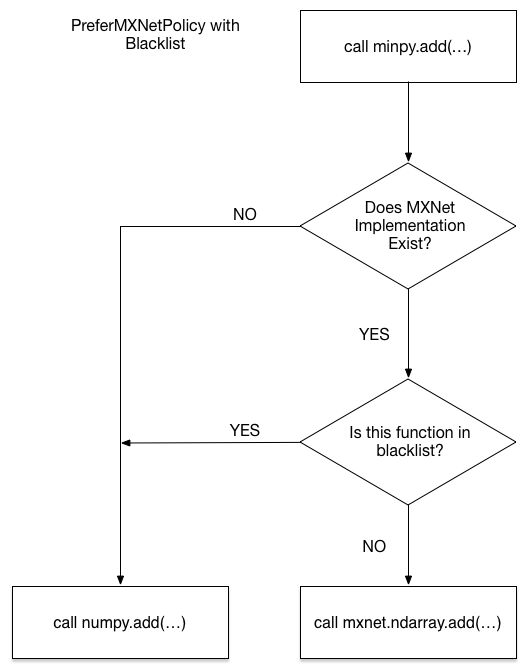# Transparent Fallback¶

This part of tutorial is also available in step-by-step notebook version on github. Please try it out!

## Concept of transparent fallback¶

Since MinPy fully integrates MXNet, it allows you to use GPU to speed up your algorithm with only minor change, while keeping the familia NumPy syntax.

However, NumPy is a giant library with many of operators, each may have different calling conventions with different parameters. MXNet’s GPU operators are only a subset of them. Therefore, it is inevitable that you may use some functions that are currently missing on the GPU side.

To solve this problem, MinPy designed a policy system to determine which implementation shoud be applied, consisted of build-in policies in minpy.dispatch.policy (also aliased in minpy root):

• PreferMXNetPolicy() [Default]: Prefer MXNet. Use NumPy as a transparent fallback, which wil be discussed below.
• OnlyNumPyPolicy(): Only use NumPy operations.
• OnlyMXNetPolicy(): Only use MXNet operations.
• BlacklistPolicy(): Discussed below.

The default policy PreferMXNetPolicy gracefully adopts the NumPy implementation once the operator is missing on GPU side, and handles the memory copies among GPU and CPU for you, illustrated with the following chart:PreferMXNetPolicy

The code below will prove this for you.

In :

import minpy.numpy as np
# First turn on the logging to know what happens under the hood.
import logging
logging.getLogger('minpy.array').setLevel(logging.DEBUG)

# x is created as a MXNet array
x = np.zeros((10, 20))

# cosh is currently missing in MXNet's GPU implementation.
# So x will fallback to a NumPy array, so you will see a
# logging like "Copy from MXNet array to NumPy array...", then
# NumPy's implementation of cosh will be called to get the
# result y as a NumPy array. But you don't need to worry
# about the memory copy from GPU -> CPU
y = np.cosh(x)

# log has MXNet's GPU implementation, so it will copy the
# array y from NumPy array to MXNet array and you will see
# a logging like "Copy from NumPy array to MXNet array..."
# Once again, you don't need to worry about it. It is transparent.
z = np.log(y)

# Turn off the logging.
logging.getLogger('minpy.array').setLevel(logging.WARN)

I1110 11:11:21 12022 minpy.array:_synchronize_data:423] Copy from MXNet array to NumPy array for Array "4580105904" of shape (10L, 20L).
I1110 11:11:21 12022 minpy.array:_synchronize_data:429] Copy from NumPy array to MXNet array for Array "4580229360" of shape (10, 20).


However, there are a few of NumPy functions cannot work properly even in the PreferMXNetPolicy, due to the difference between NumPy and MXNet interface. Here is one example with different parameter types:

In :

# Uner PreferMXNetPolicy, np.random.normal will redirect to MXNet's implementation
# but it does not support mu and sigma to be arrays (only scalar
# is supported right now).
import minpy.numpy as np
def gaussian_cluster_generator(num_samples=10000, num_features=500, num_classes=5):
mu = np.random.rand(num_classes, num_features)
sigma = np.ones((num_classes, num_features)) * 0.1
num_cls_samples = num_samples / num_classes
x = np.zeros((num_samples, num_features))
y = np.zeros((num_samples, num_classes))
for i in range(num_classes):
# this line will occur an error
cls_samples = np.random.normal(mu[i,:], sigma[i,:], (num_cls_samples, num_features))
x[i*num_cls_samples:(i+1)*num_cls_samples] = cls_samples
y[i*num_cls_samples:(i+1)*num_cls_samples,i] = 1
return x, y

gaussian_cluster_generator(10000, 500, 5)

---------------------------------------------------------------------------
MXNetError                                Traceback (most recent call last)
<ipython-input-2-3e8f056001e5> in <module>()
16     return x, y
17
---> 18 gaussian_cluster_generator(10000, 500, 5)

...

/Users/ATlaS/Library/PyEnvs/minpy/lib/python2.7/site-packages/mxnet-0.7.0-py2.7.egg/mxnet/base.pyc in check_call(ret)
75     """
76     if ret != 0:
---> 77         raise MXNetError(py_str(_LIB.MXGetLastError()))
78
79 if sys.version_info < 3:

MXNetError: Invalid Parameter format for loc expect float but value='<mxnet.ndarray.NDArray object at 0x11101d190>'


What that means is we must control dispatch at a finer granularity. We design another blacklist machinism for you. The operator in the blacklist will fallback to its numpy implementaiton and the content of blacklist will be prepared when you install MinPy automatically. This will solve most of these problems.

The procedure of function call under PerferMXNetPolicy will become:Blacklist

The default blacklist is generated by testing the calls in this file. The test may not be complete, therefore you can run your code iteratively and generate a customized blacklist under AutoBlacklistPolicy:

In [ ]:

import minpy
p = minpy.AutoBlacklistPolicy(gen_rule=True, append_rule=True)
set_global_policy(p)

# under AutoBlacklistPolicy, operators throwing exception will be
# added into the blacklist, then MinPy will call the NumPy
# implementation next time to avoid this kind of exception.
with p:
gaussian_cluster_generator(10000, 500, 5)

# this will not occur error afterwards
gaussian_cluster_generator(10000, 500, 5)


Do check “Pitfalls when working together with NumPy” for known issues. If you encounter another, please raise an issue in our github!